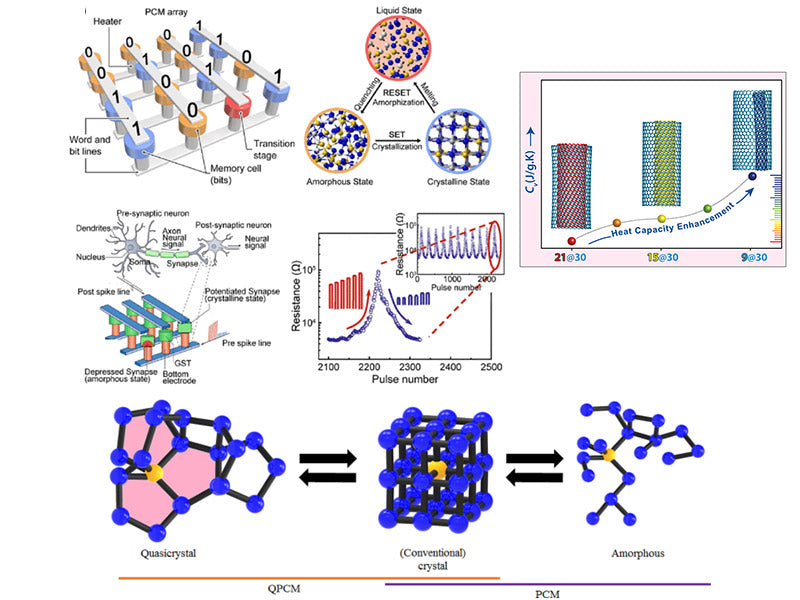Paraffin is generally a mixture of straight-chain alkanes. Therefore, when using molecular dynamics to simulate paraffin-based PCM(phase change materials), the model of the PCM system is generally composed of simple alkanes or a mixture of alkanes. According to the requirements of parameters such as the PCM phase transition temperature of the power battery thermal management system, alkanes with a melting point of 35-50 °C were selected for molecular dynamics simulation. First, the pure PCM system composed of n-nonadecane (C19H40), n-eicosane (C20H42), n-hexadecane (C21H44) and n-docosane (n-docosane, C22H46) was established respectively, where the PCM configurations based on n-nonadecane and n-docosane are shown in Figure 1. Each system is composed of 40 molecular chains, the structure is amorphous, and the cubic box is used.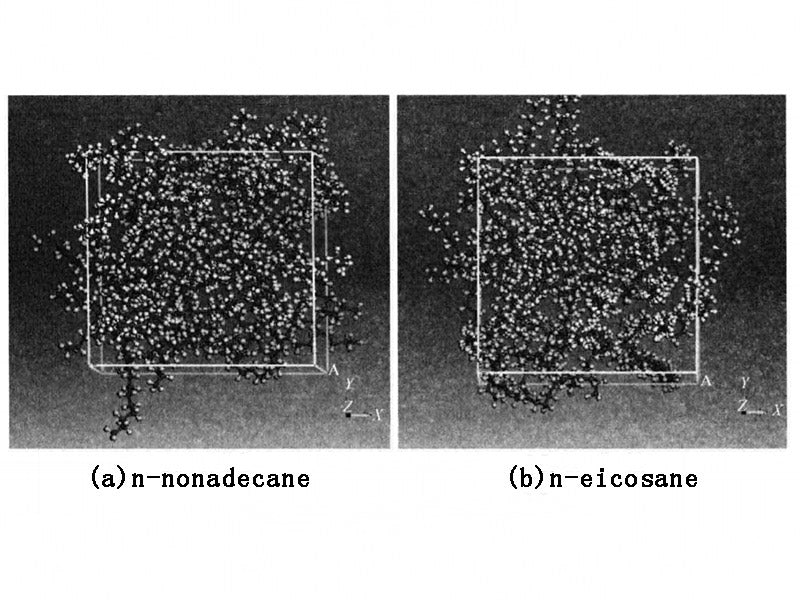Figure 1 - Amorphous structure of PCM system

Specific heat (including specific heat capacity at constant pressure and specific heat capacity at constant volume) is used as the main thermophysical parameter of PCM. In molecular dynamics simulation, the specific heat capacity at constant pressure (Cp) can be calculated by formula (1-1):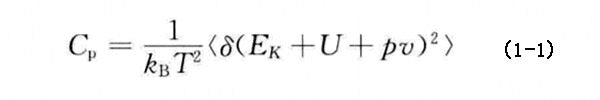where kB stands for Boltzmann constant; T stands for temperature; EK and U stand for kinetic energy and potential energy, respectively; p and v stand for system pressure and volume, respectively; <> stands for ensemble average.

Figure 2 shows the variation of the total energy and constant pressure specific heat capacity of each PCM system based on n-alkane with temperature. With the increase of the number of alkane C atoms and the simulation time, the total energy and constant pressure specific heat capacity of each PCM system showed good consistency during the heating and cooling process. Van Miltenburg et al. have given the experimental test values of the specific heat capacity at constant pressure in the temperature range of 10~390K for n-nonadecane and n-eicosane. Among them, the Cp value of nonadequate increases from 1.82kJ·kg-1·K-11 at 280K to 2.41kJ·kg-1·K-1 at 360K, the Cp value of eicosine increases from 1.68kJ·kg-1·K-1 at 280K to 2.41kJ·kg-1·K-1 at 360K, and the simulation results are very close to the experimental results. When the temperature is lower than the PCM phase transition temperature, the constant pressure specific heat capacity of the simulated n-nonadecane and n-eicosane does not differ by more than 20% from the experimental value, and when the temperature is higher than the PCM phase transition temperature, it does not exceed 9.5%. The main reasons for the deviation may include the purity of the alkane used in the experiment, the accuracy of the experimental instrument and the experimental conditions, or the simplification of the model in the molecular dynamics simulation and the adaptability of the force field. However, the closeness of the simulated and experimental values also indicates that the amorphous PCM structure is established, and the microscopic mechanism of the change of the macroscopic thermophysical properties of the PCM can be recognized and understood.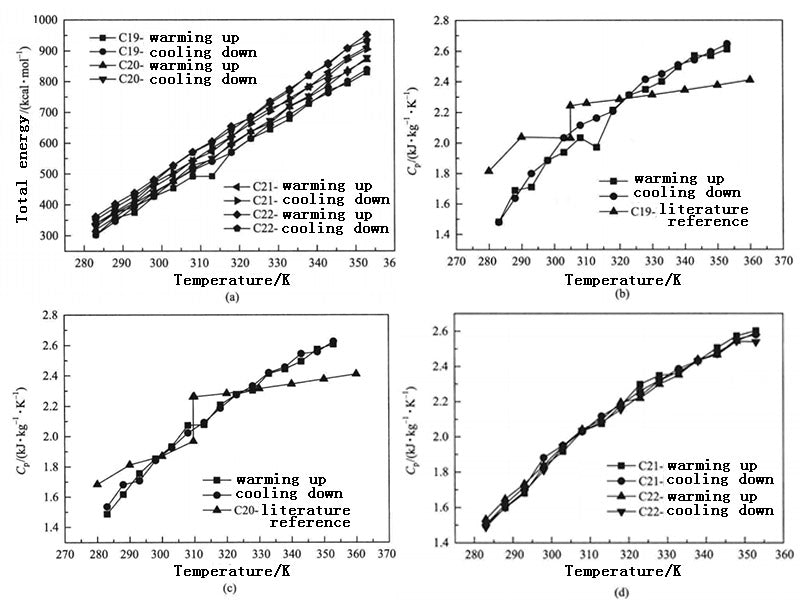Figure 2 - Total energy and constant pressure specific heat capacity as a function of temperature for different PCM systems

Figure 3 shows the thermal conductivity of the PCM system composed of n-docosane under normal pressure as a function of temperature. When the system temperature is 283K, 288K, 293K, 298K, 303K, 308K, 313K, 318K, 323K and 328K, the corresponding thermal conductivity calculated is 0.06W·m-1·K-1, 10.12W·m-1·K-1, 0.11W·m-1·K-1, 0.23W·m-1·K-1, 0.33W·m-1·K-1, 0.37W·m-1·K-1, 0.23W·m-1·K-1, 0.24W·m-1·K-1, 0.52W·m-1·K-1 and 0.49W·m-1·K-1. The thermal conductivity of paraffin-based PCMs given in the literature and in the range of 0.1~0.4W·m-1·K-1. Therefore, when the temperature is between 288~318K, it can be considered that the thermal conductivity calculated by molecular dynamics is close to the range of macroscopic experimental data, and the calculation results are meaningful.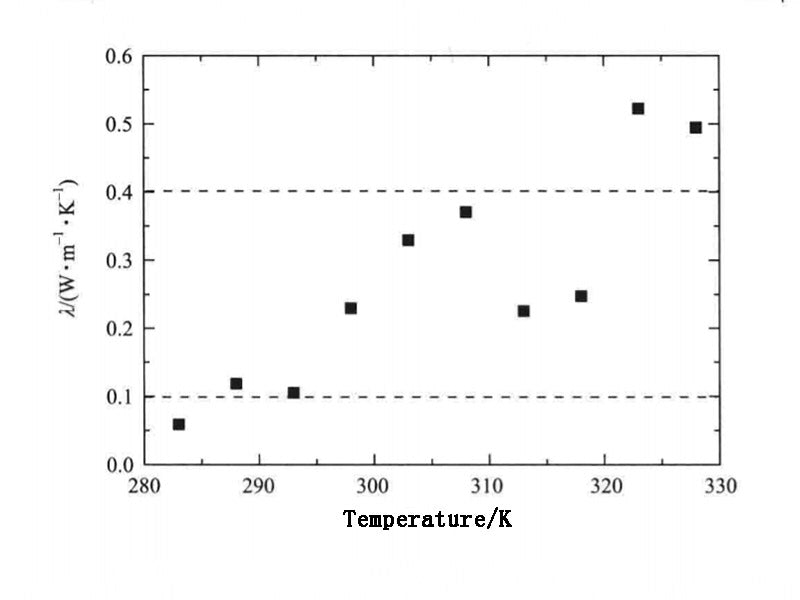Figure 3 - Thermal conductivity of n-docosane

The thermal conductivity of calcined hydrocarbons is also affected by pressure. The literature gives the variation of thermal conductivity of several calcined hydrocarbons that are liquid at room temperature from 0.1 to 250 MPa under pressure. The results show that with the increase of pressure, the thermal conductivity of calcined hydrocarbons increases. For n-docosane, the PCM system that is solid at room temperature, its self-diffusion coefficient and thermal conductivity change with pressure are shown in Figure 4. When 0.1MPa, the thermal conductivity obtained by the three calculations are 0.233W·m-1·K-1, 0.216W·m-1·K-1, 0.228W·m-1·K-1, respectively; when 0.5MPa, they are 0.150W·m-1·K-1, 0.136W·m-1·K-1, 0.186W·m-1·K-1 respectively. It is not difficult to see that with the increase of system pressure, the self-diffusion coefficient and thermal conductivity both show a slight downward trend. The reason is that the increase of pressure limits the stretching and twisting of the n-docosane molecular chain. In addition, when the pressure increased to 0.5MPa, the thermal conductivity decreased little. In practical application, the influence of the pressure change in the PCM system caused by the sealing on the thermal conductivity of the PCM can be ignored.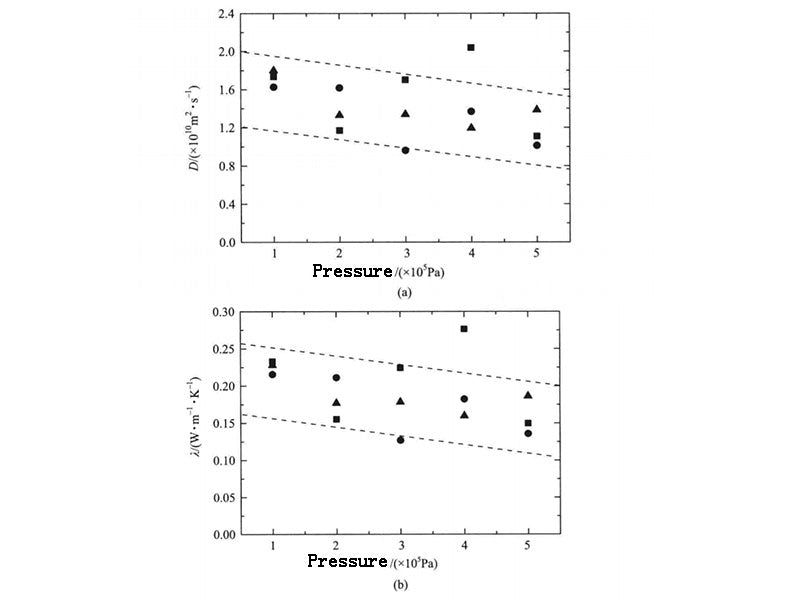Figure 4 - Self-diffusion and thermal conductivity of n-docosine under different pressures